# 纹理映射（Texture Mapping）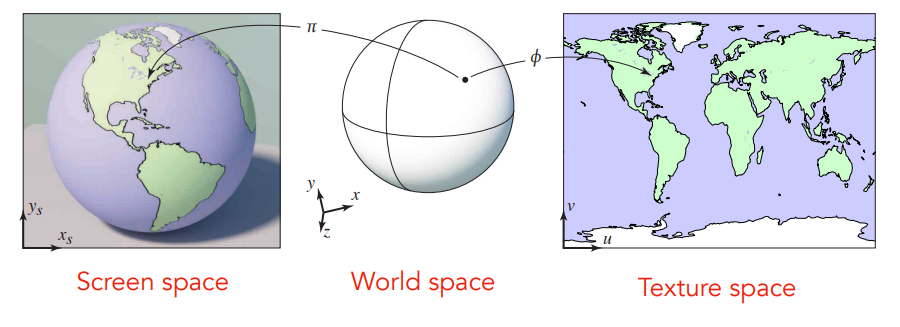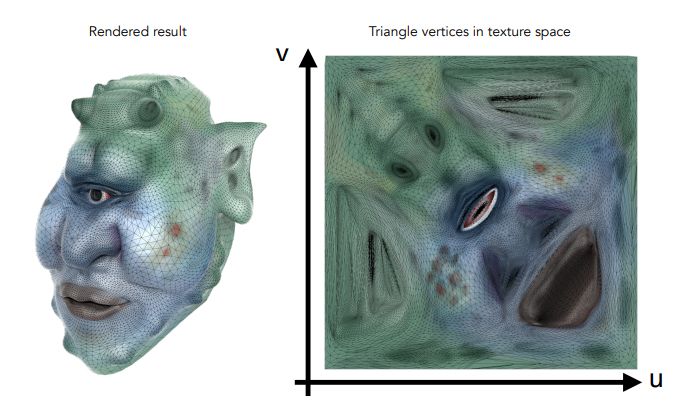## 球形贴图（Spherical Map）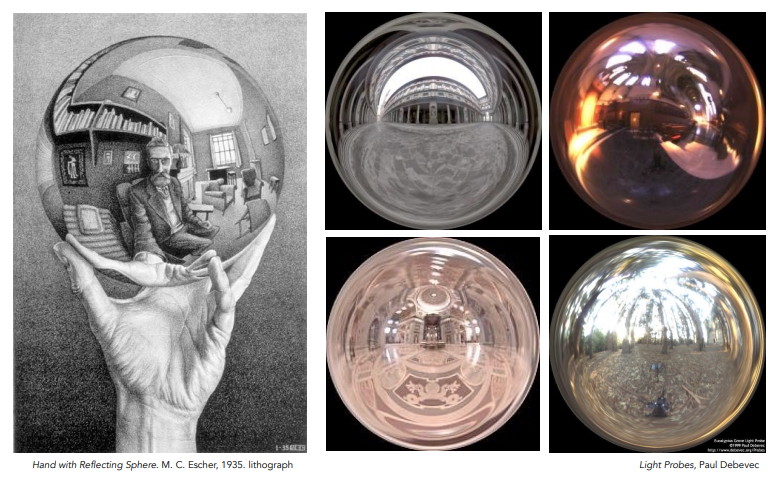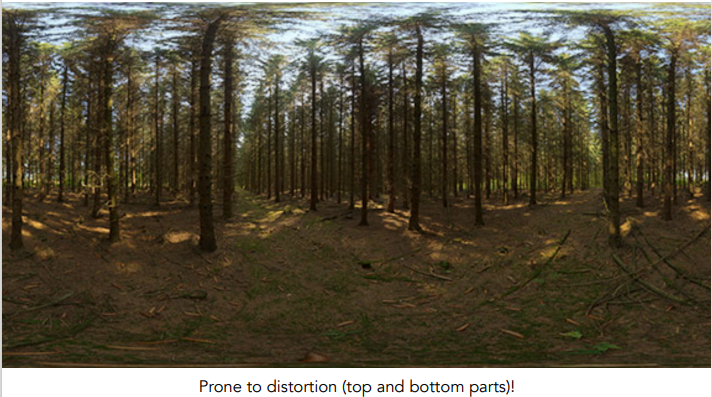1. 假设一个单位球包围了物体中心，当物体中心看向物体表面某个位置 $$(x,y,z)$$ 时，从中心朝这个位置发出一条射线，此时射线会与单位球相交于某点。
2. 根据射线与单位球体的交点坐标 $$(x_o,y_o,z_o)$$​，推算出交点所在的偏航角和俯仰角 $$(yaw,pitch)$$​，然后来映射成在球形贴图对应的 $$(u,v)$$​​​ 坐标点。

## 立方体贴图（Cube Map）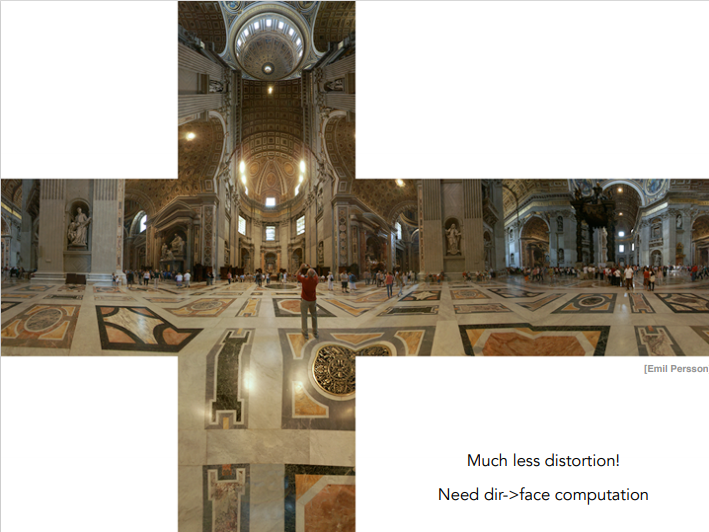1. 假设一个单位立方体包围了物体中心，当物体中心看向物体表面某个位置 $$(x,y,z)$$ 时，从中心朝这个位置发出一条射线，此时射线会与单位立方体相交于某点。
2. 根据视线与单位立方体的交点坐标 $$(x_o,y_o,z_o)$$，在$$x_o$$$$y_o$$$$z_o$$中取绝对值最大的那个分量，根据它的符号来判定来确定要映射在哪一个面。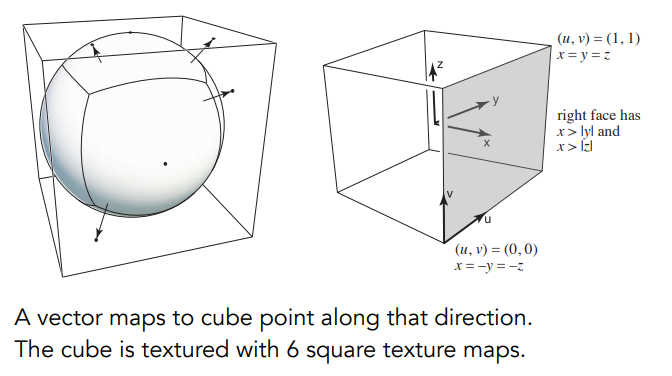1. 确定映射在第几个面后，剩余另外两个分量便是来映射成在第几张立方体贴图中的 $$(u,v)$$ 坐标点。

# 纹理走样问题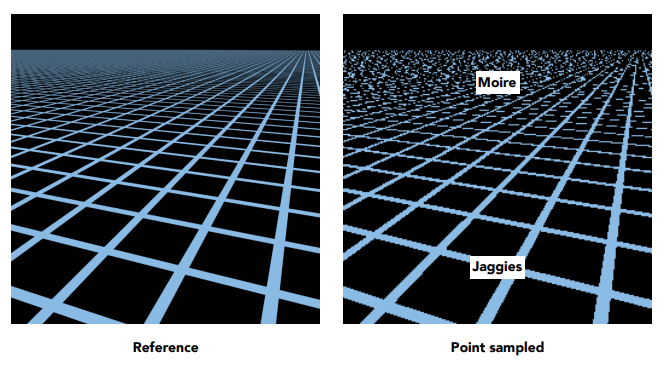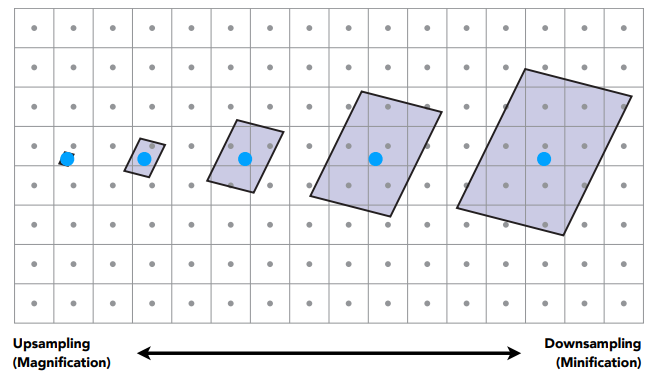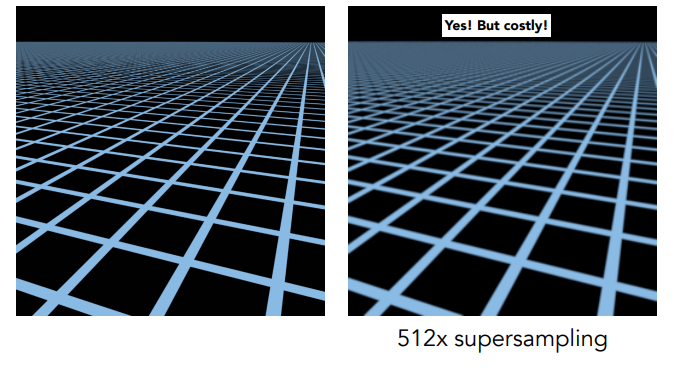## Mipmap

Mipmap技术则可以让纹理采样进行快速的近似正方形范围查询，它需要预先提供多层分辨率各不同（每层纹理长宽都比上层缩小一半）的纹理（用D表示它们的层级）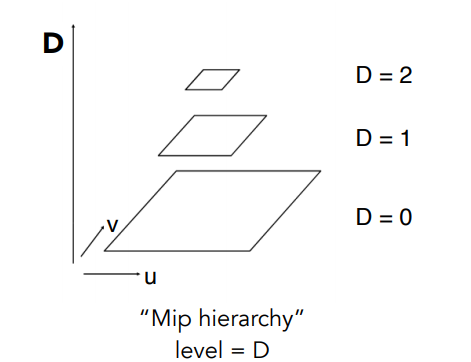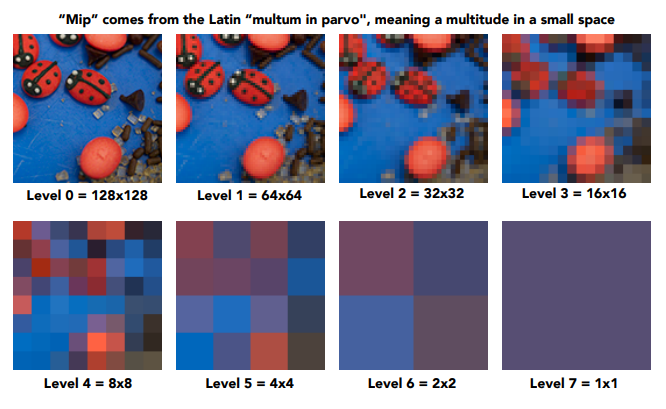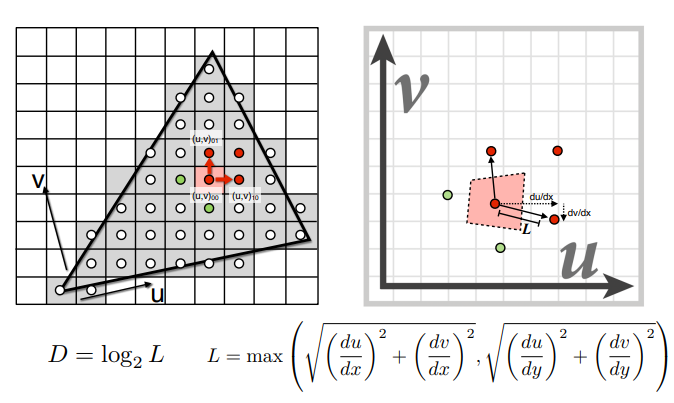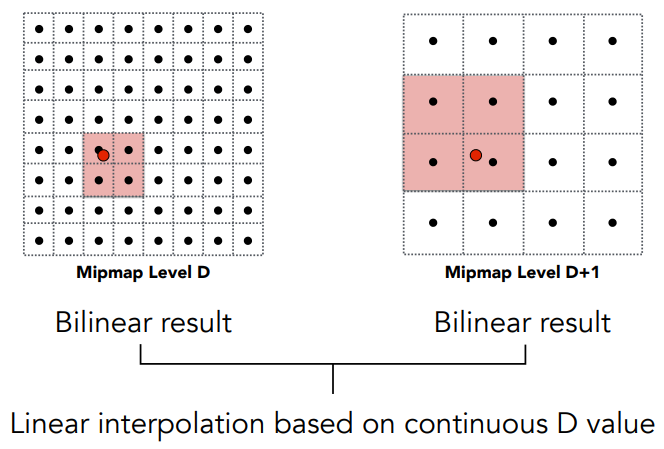## 各向异性过滤（Ripmap）

Mipmap的一个缺点是，它只适合近似正方形范围查询，当一个屏幕像素点对应的纹素范围是别的形状（尤其长条形状）时，很容易导致近似正方形覆盖的区域比实际覆盖区域大得多，从而造成过度模糊现象（例如下图中远处的格子已经模糊地看不见黑色区域）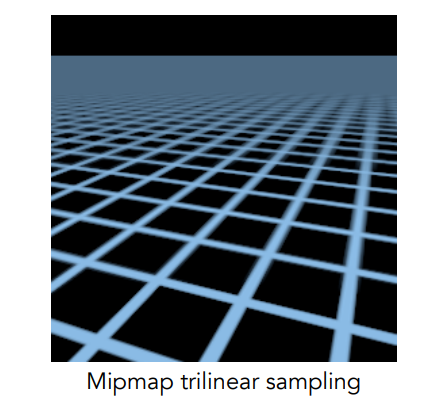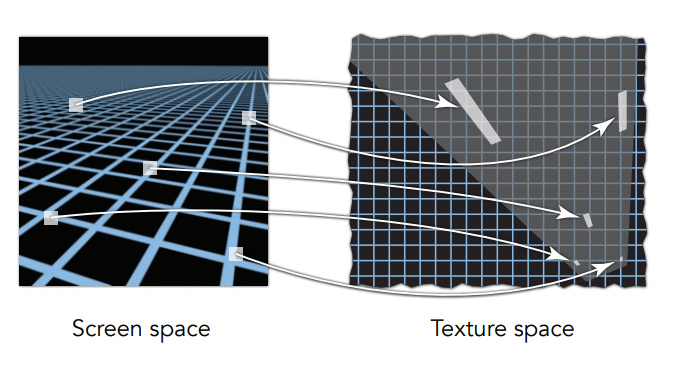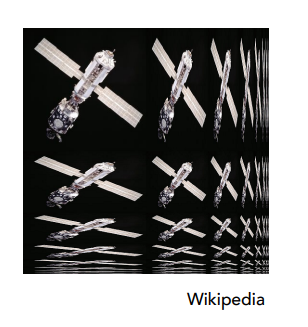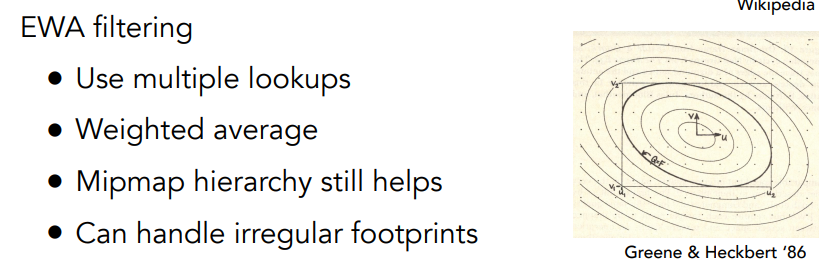# 纹理应用技术（Texture Application）

## 天空盒（SkyBox）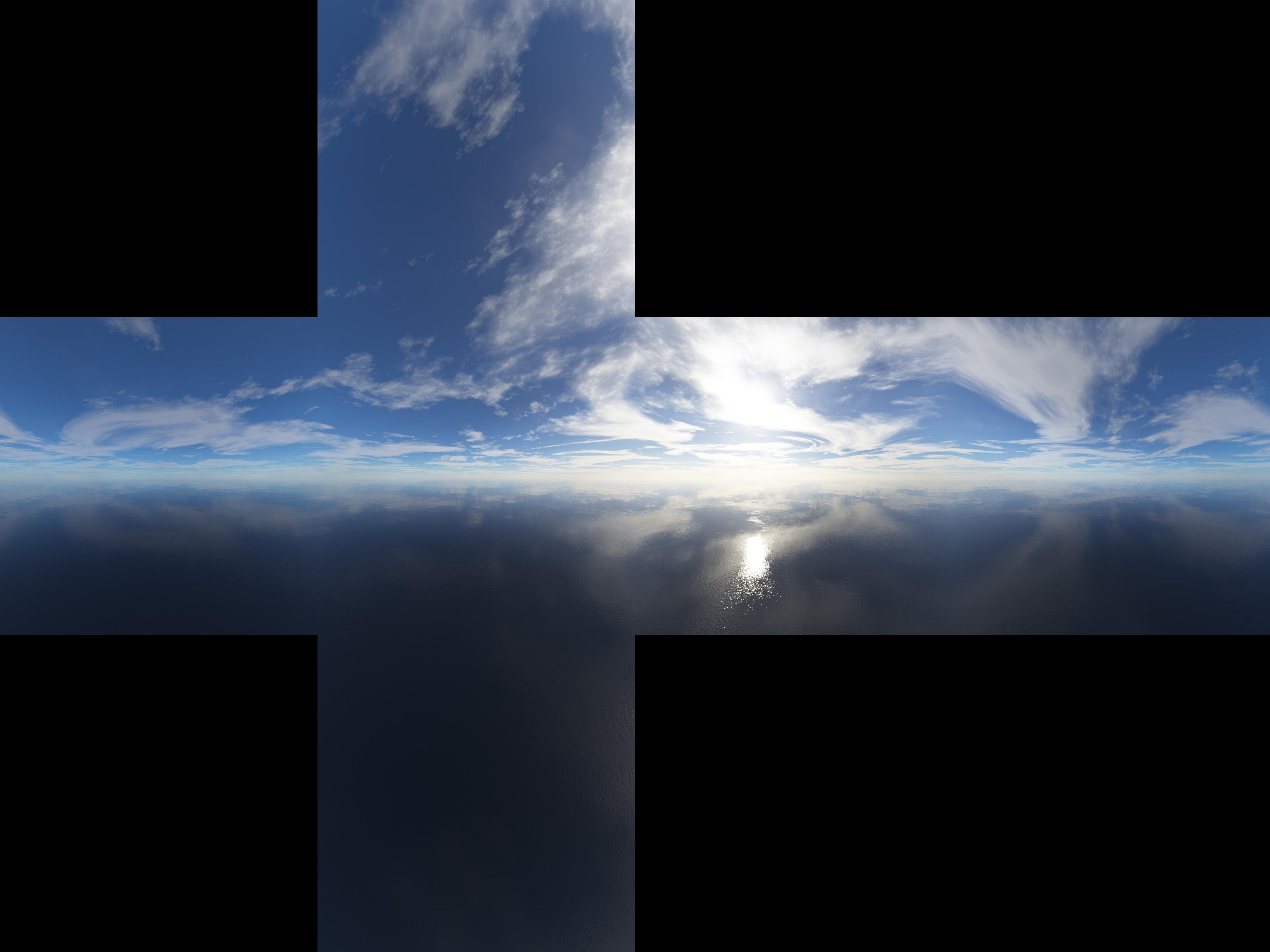1. 先准备6个面的天空背景制成 Cube Map。
2. 将一个不会旋转、大小随意的立方体物体始终罩在摄像机的周围（让摄像机始终位于这个立方体的中心位置）。

• 天空盒渲染应该放在最后的渲染顺序，可以减少很多像素overdraw开销（放在最后的渲染顺序可以直接丢弃大量像素，而不必对这些迟早要丢弃的像素进行着色）

## 环境映射（Environment Mapping）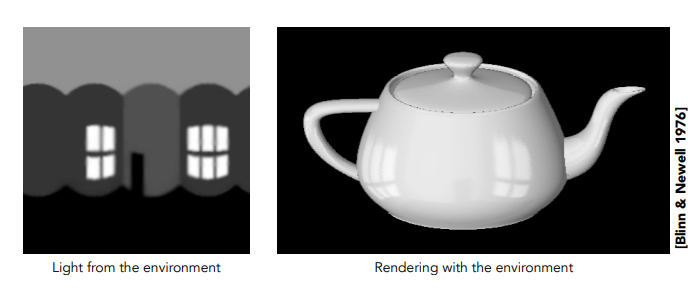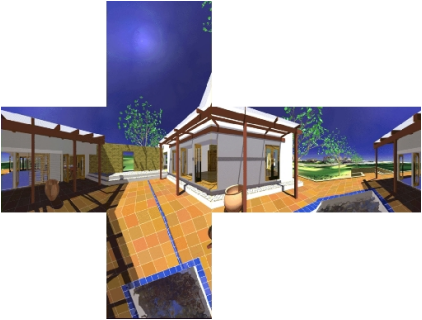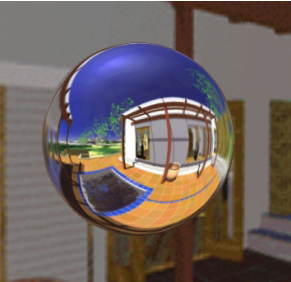1. 设置6个摄像机位于物体中心，每帧分别朝六个方向渲染得到的图像输出到立方体贴图里对应的面上。

• 一般的环境贴图并不需要高精度的环境光信息，因此可以使用分辨率更低的环境贴图（这样生成环境贴图的开销也会减少不少）。

## 光照贴图（Light Map）

1. 烘培（Bake）：预先计算这些复杂的光照，并保存进一个专门存放光照计算结果的光照贴图（Light Map）。
2. 当物体在着色的时候，不仅采样正常纹理+Light Map，便可以让物体拥有高质量的着色。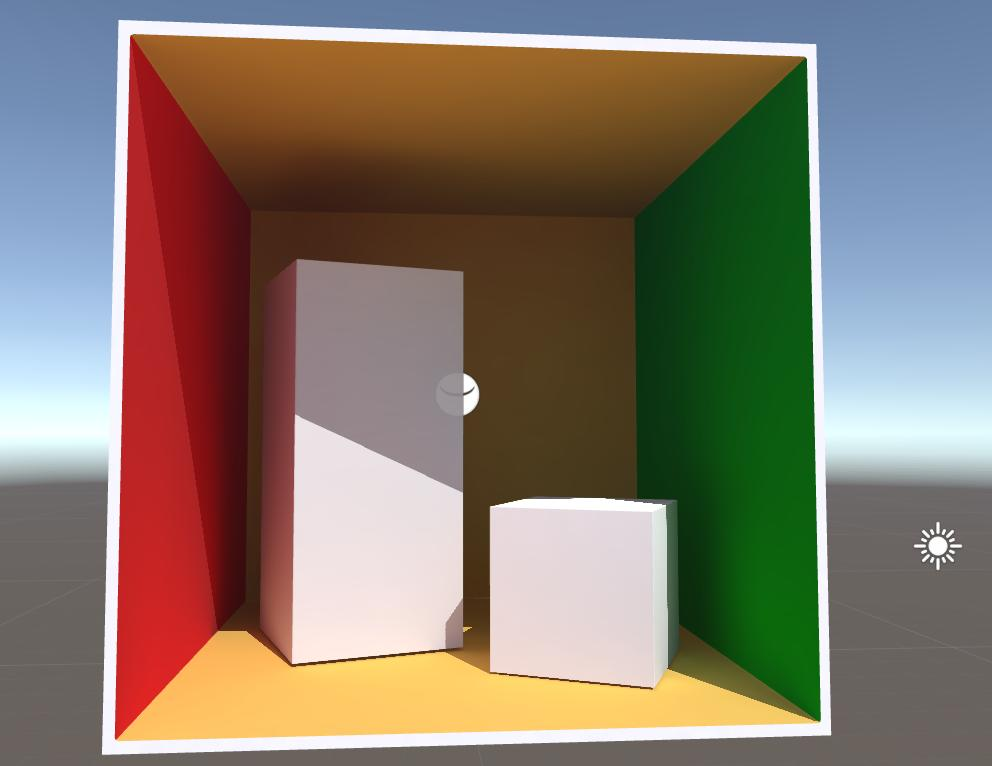• 烘培光照，可以减少大量运行时的开销。

• 光照是静态的，不能动态变化（预计算特点），因此只适用于静态物体。
• 烘培（即预计算光照）的用时是漫长的，需要开发人员的耐心 😃
• 若场景同时包含静态物体和动态物体，需要考虑静态光照与对动态光照的结合算法。

## 环境光遮蔽贴图（Ambient Occlusion Texture Map）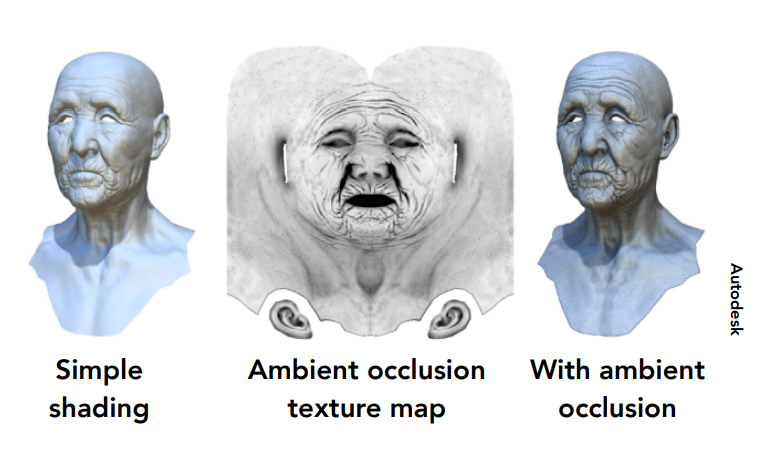## 凹凸映射（Bump Mapping）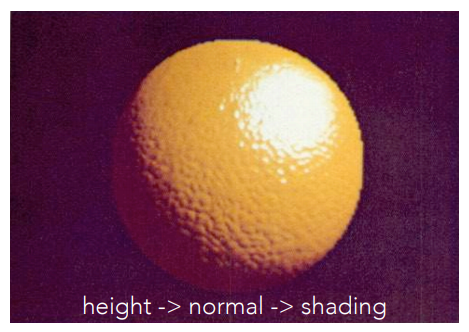### 高度贴图（Height Map）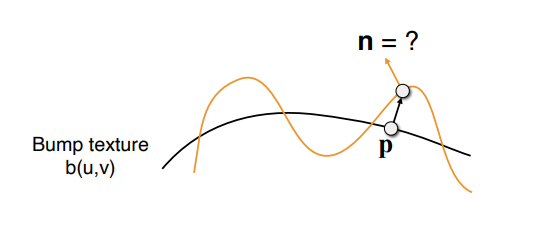1. 先计算出p点上u、v方向的切线值：

$$\frac{dp}{du} = c1* [H(u+1,v)-H(u,v)]$$​​​​

$$\frac{dp}{dv} = c2* [H(u,v+1)-H(u,v)]$$​​​​​

1. $$p$$ 点的法线（切线空间下）为：

$$n_{tangent}(p) = Normalize(-\frac{dp}{du},-\frac{dp}{dv},1)$$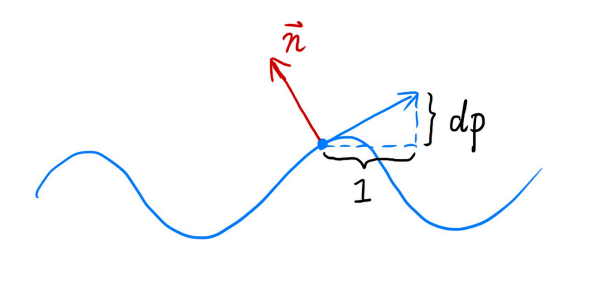### 模型空间的法线贴图（Object-Space Normal Map）

$$pixel=(n+1)/2$$

$$n=pixel∗2−1$$

### 切线空间的法线贴图（Tangent-Space Normal Map)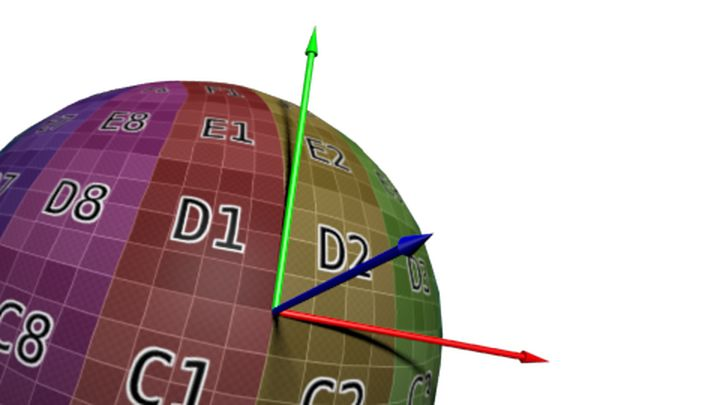1. $$B = N \times T$$ ，其中 $$N$$$$T$$ 分别为顶点上的原始的法线、切线（tangent），$$B$$ 则称为副切线（bitangent）。

2. $$n = [T\ B\ N]\cdot n_{tangent}$$

• 自由度高、可重用：模型空间下的Normal Map记录的是绝对法线信息，仅可以用于创建它的那个模型；而切线空间下的Normal Map记录的是相对法线信息，可用于任何朝向的任何模型/面。
• 可进行UV动画：可以移动一个纹理的UV坐标来实现凹凸移动的效果。UV动画在水或者火山熔岩这种类型的物体长经常用到。
• 可压缩：由于Tangent-Space Normal Map中法线的Z方向总是正方向的，因此我们可以仅存储XY方向，而推导得到Z方向（通过向量标准化）。

• 切线空间的法线贴图需要计算额外的空间变换：在计算光照时，需要统一各种方向向量所在的坐标空间，而法线贴图中存储的法线是切线空间下的方向，因此需要额外计算（模型空间下的法线贴图则不需要）。

1. 切线空间下进行光照计算，需要把光照方向、视角方向变换到切线空间下（性能上较优，常用）
2. 世界空间下进行光照计算，需要把采样得到的法线方向变换到世界空间下，再和世界空间下的光照方向和视角方向进行计算。（通用性上较优）

### 视差贴图（Parallax Map/Offset Map）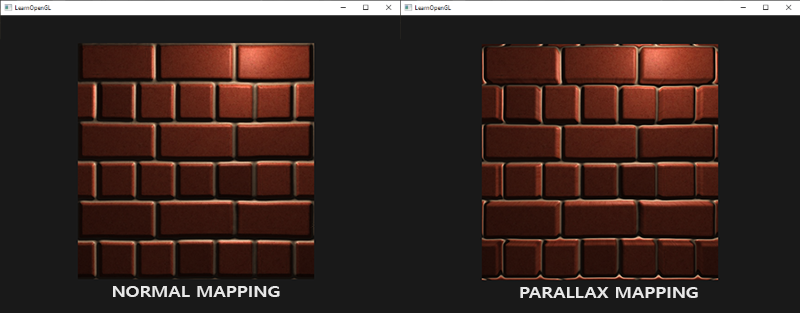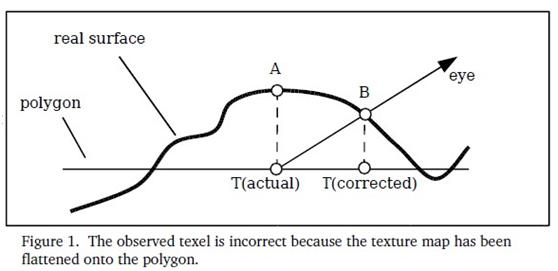Parallax算法的解决方法是：

1. 从原先直接看到的点 $$T_a$$​​​ 往视角方向 $$V$$​ 位移一定距离（与$$T_a$$​的高度成正比），得到该位移的水平偏移 $$offset$$​ （将是个二维向量）。​​​

$$offset = c\cdot H(u,v)\cdot\frac{[V_x \ V_y]^T}{V_z}$$​​

1. 那么更加近似正确结果的点应该是：

$$T_b = T_a+offset$$​​​

1. 将点用于法线贴图的查询，那么 $$NomalMap(T_b)$$​ 即为所见法线。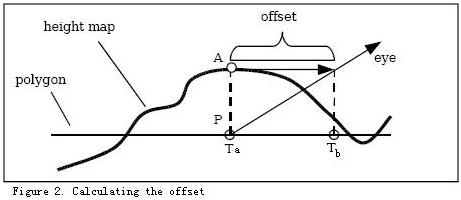• 无论是哪个角度看向使用Parallax Map的物体，都能有近似正确凹凸的效果

• 需要高度贴图，这意味着要不使用高度贴图+法线贴图的组合（占额外显存空间），要不单纯靠高度贴图计算法线（计算量多些）

### 浮雕贴图（Relief Map）

Relief Map又叫 浮雕纹理 ,是对 Parallax 的进一步精确。如果说Parallax只是根据视角方向在切线空间下的投影和高度图作近似的偏移，那么Relief Map则是以找到正确点B点进行采样为目标，具体分为两步：

1. 通过步进法（Linear Search）找到交点的大致范围：从观察点朝视角方向步进一定距离（方向不变）进行一次采样，若发现采样的高度高于视角线高度，则意味着该点为范围的一端（观察点为另一端）：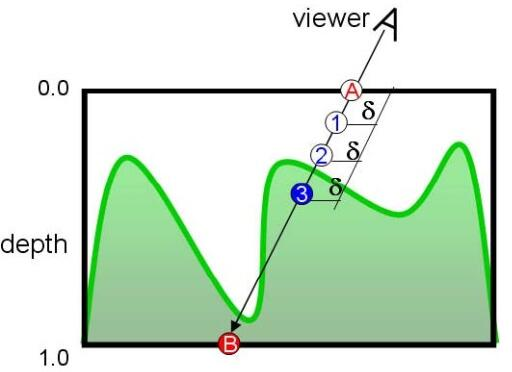1. 通过二分法（Binary Search）进一步找到交点：在确定大致范围后，步进距离每次减半（方向可变），直到逼近目标值：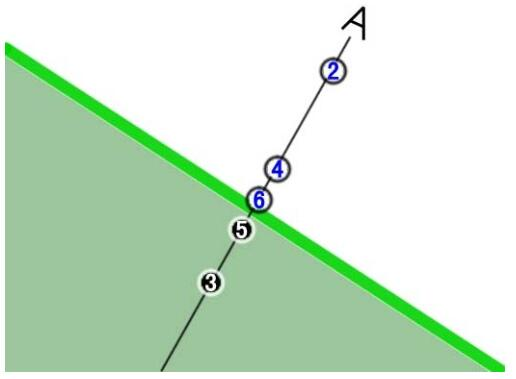• 相比与Parallax Map，不同角度看向物体都有更加精确的凹凸效果。

• 和Parallax Map一样，需要高度贴图
• 多轮迭代寻找采样点，计算非常耗时

## 位移映射（Displacement Mapping）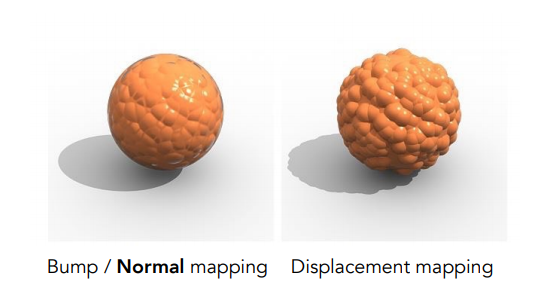• 修改顶点位置，需要重新计算由此分出来的新三角形
• 产生更多数量的三角形，开销大大增加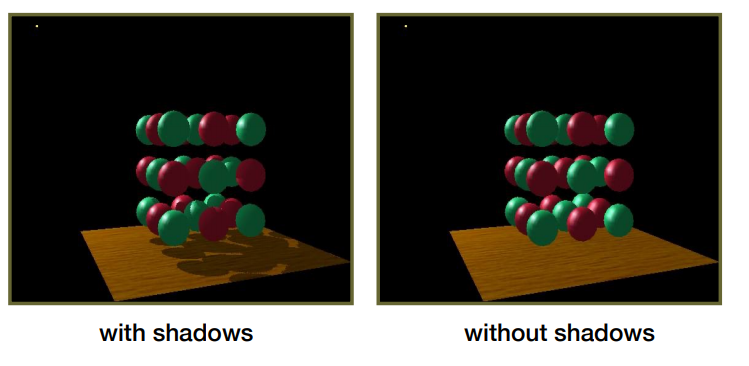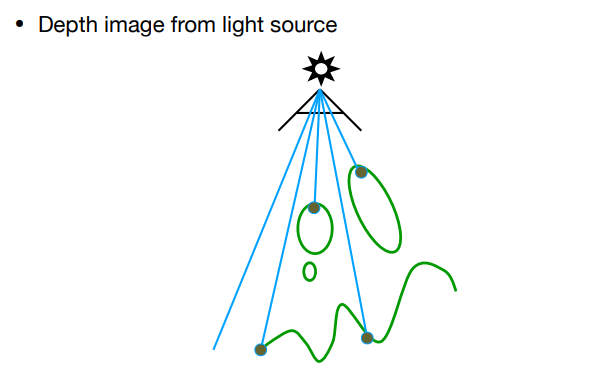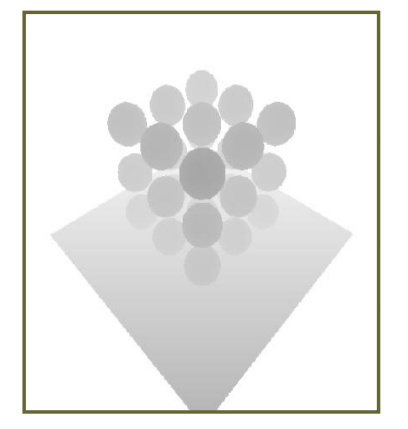1. 主摄像机需要渲染每个像素时，通过光源摄像机的MVP变换，便能得到该像素点在光源摄像机屏幕空间中对应的位置$$(x',y',z')$$​；接着深度值 $$z$$​ 和阴影贴图（Shadow Map）用$$(x',y')$$​采样得到的遮挡深度做深度比较： 若深度 $$z$$​ 大于对应遮挡深度（意味着该像素的光被遮挡），这时就可以对该像素降低明亮度。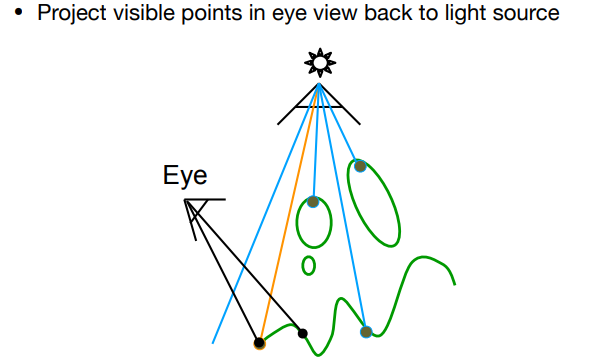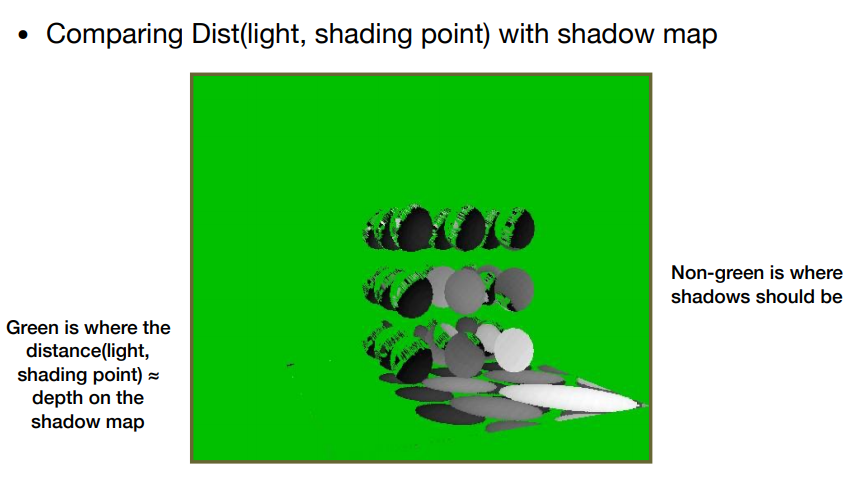## 3D纹理（3D Textures）

### 3D柏林噪声（3D Perlin Noise）生成纹理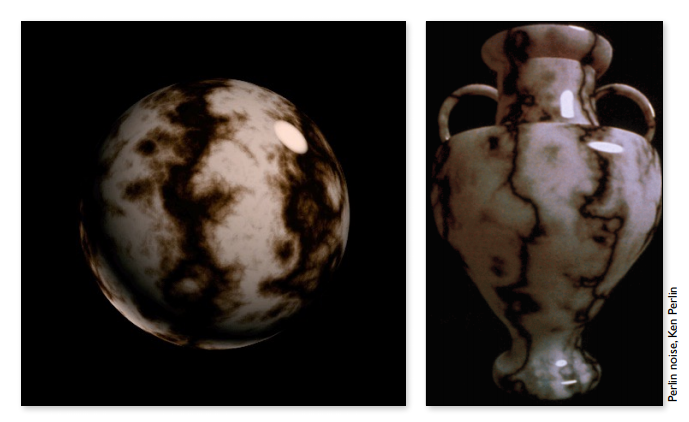### 体积渲染（Volume Rendering）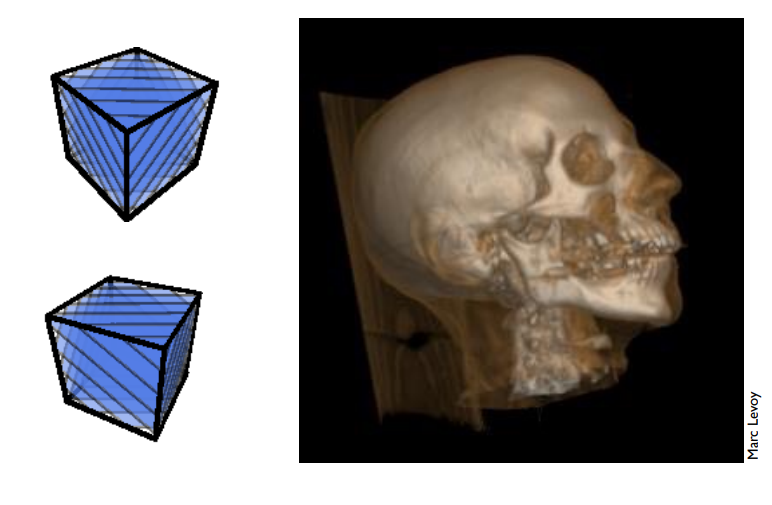# 参考

posted @ 2021-08-11 00:17  KillerAery  阅读(1847)  评论(0编辑  收藏  举报